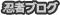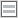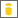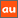# 0除算に関するアナウンスメントを公開しています

The purpose of this site is to publish announcements on the theory of division by zero

## Announcement 282: The division by zero z/0=0 on the Second Birthday

Institute of Reproducing Kernels
Kawauchi-cho, 5-1648-16,
Kiryu 376-0041, Japan
E-mail: kbdmm360@yahoo.co.jp
February 2, 2016

Abstract: In this announcement, we would like to request some formal acceptance of the division by zero as the definite results and  to spread over the world the elementary and fundamental mathematics  on the occasion of the second birthday of the division by zero starting at February 2, 2014.

Introduction
By a natural extension of the fractions
\begin{equation}
\frac{b}{a} \tag{1.1}
\end{equation}for any complex numbers $a$ and $b$, we found the result, for any complex number $b$
\begin{equation}
\frac{b}{0}=0, \tag{1.2}
\end{equation}incidentally in  by the Tikhonov regularization for the Hadamard product inversions for matrices, and we discussed their properties and gave several physical interpretations on the general fractions in  for the case of real numbers. The result is a very special case for general fractional functions in .

The division by zero has a long and mysterious story over the world $($see, for example, Google site with the division by zero$)$ with its physical viewpoints since the document of zero in India on AD 628,  however, Sin-Ei, Takahasi  $($$)$ established a simple and decisive interpretation $($1.2$)$ by analyzing the extensions of fractions and by showing the complete characterization for the property $($1.2$)$:

Proposition. Let F be a function from ${\bf C }\times {\bf C }$ to ${\bf C }$ satisfying
\begin{equation}
F (b, a)F (c, d)= F (bc, ad)
\end{equation}for all $a, b, c, d \in {\bf C }$, and
$$F (b, a) = \frac {b}{a }, \quad a, b \in {\bf C }, a \ne 0.$$Then, we obtain, for any $b \in {\bf C }$
$F (b, 0) = 0.$

We thus should consider, for any complex number $b$, as  $($1.2$)$; that is, for the mapping
\begin{equation}
w = \frac{1}{z},
\end{equation} the image of $z=0$ is $w=0$. This fact seems to be a curious one in connection with our well-established popular image for the  point at infinity on the Riemann sphere. Therefore, the division by zero will give great impacts to complex analysis and to our idea for the space and universe.
However, the division by zero $($1.2$)$ is now clear, indeed, for the introduction of $($1.2$)$, we have several independent approaches as in:
1) by the generalization of the fractions by the Tikhonov regularization or by the Moore-Penrose generalized inverse,
2) by the intuitive meaning of the fractions $($division$)$ by H. Michiwaki,
3) by the unique extension of the fractions by S. Takahasi,   as in the above,
4) by the extension of the fundamental function $W = 1/z$ from ${\bf C} \setminus \{0\}$ into ${\bf C}$ such that $W =1/z$ is a one to one and onto mapping from ${\bf C} \setminus \{0\}$ onto ${\bf C} \setminus \{0\}$ and the division by zero $1/0=0$ is a one to one and onto mapping extension of the function $W =1/z$ from  ${\bf C}$ onto ${\bf C}$,
and
5) by considering the values of functions with the mean values of functions.
Furthermore, in $($$)$ we gave the results in order to show the reality of the division by zero in our world:
A) a field structure  containing the division by zero --- the Yamada field ${\bf Y}$,
B)  by the gradient of the $y$ axis on the $(x,y)$ plane --- $\tan \frac{\pi}{2} =0$,
C) by the reflection $1/\overline{z}$ of $z$ with respect to the unit circle with center at the origin on the complex $z$ plane --- the reflection point of zero is zero,
and
D) by considering rotation of a right circle cone having some very interesting
phenomenon  from some practical and physical problem --- EM radius.
See also  for the relationship between fields and the division by zero, and the importance of the division by zero for computer science.

Meanwhile,  J. P.  Barukcic and I.  Barukcic $($$)$ discussed recently the relation between the division $0/0$ and special relativity of Einstein.  Furthermore,  Reis and Anderson $($[7,8]$)$ extends the system of the real numbers by defining division by zero.
For our results, see the survey style announcements 179,185,237,246, 247,250 and 252 of the Institute of Reproducing Kernels $($[11,12,13,14,15,16,17,18]$)$.
At this moment, the following theorem may be looked as the fundamental theorem of the division by zero:

Theorem $($$)$. Any analytic function takes a definite value  at an  isolated singular point  with a natural meaning.

The following corollary shows  how to determine the value of an analytic function at the singular point; that is,  the value is determined from the regular part of the Laurent expansion:

Corollary 1. For an isolated singular point $a$ of an analytic function $f(z)$, we have the Cauchy integral formula
$$f(a) = \frac{1}{2\pi i} \int_{\gamma} f(z) \frac{dz}{z - a},$$where $\gamma$ is a rectifiable simple Jordan closed curve that surrounds one time the point $a$ on a regular region of the function $f(z)$.

The essential meaning of this theorem and corollary is given by that:  the values of functions may be understood in the sense of the mean values of analytic functions.
For the importance of the division by zero, look the followings:
Impact of 'Division by Zero' in Einstein's Static Universe and ...
www.researchgate.net/.../242574738  Impact of 'Division by Zero' in Einstein's Static Universe and Newton's Equations in Classical Mechanics on ResearchGate, the professional network for.
In particular:  George Gamow $($1904-1968$)$ Russian-born American nuclear physicist and cosmologist remarked that "it is well known to students of high school algebra" that division by zero is not valid; and Einstein admitted it as the biggest blunder of his life : 1. Gamow, G., My World Line $($Viking, New York$)$. p 44, 1970

2 Results
The above statements in Introduction state clearly and definitely that:
I): The division by zero $z/0$ is possible and always $z/0=0$ by the unique extension of the division
and
II): The above results are determined as mathematics and give great impacts to not only mathematics and physics but also human beeings and our basic ideas on the universe.

3 Conclusion
Our mathematical world should declare and fix the fundamental facts I and II since AD 628.
The missing of the division by zero so long years is a great missing not only in mathematics but also in the world history. For the sake of the great missing, physics and our basic ideas on the universe led to wrong delay and wrong ideas. We should  fill the great mssing in mathematics and mathematical sciences.
Our shameful world history should close and we should open a new world. The division by zero is trivial and clear.  By the division by zero, we will be able to see a new beautiful world.

References
 J. P.  Barukcic and I.  Barukcic, Anti Aristotle - The Division Of Zero By Zero,
ViXra.org $($Friday, June 5, 2015$)$ © Ilija Barukčić, Jever, Germany. All rights reserved. Friday, June 5, 2015 20:44:59.
 J. A. Bergstra, Y. Hirshfeld and J. V. Tucker, Meadows and the equational specification of division $($arXiv:0901.0823v1[math.RA] 7 Jan 2009$)$.
 L. P.  Castro and S. Saitoh,  Fractional functions and their representations,  Complex Anal. Oper. Theory 7 $($2013$)$, no. 4, 1049-1063.
 M. Kuroda, H. Michiwaki, S. Saitoh, and M. Yamane,
New meanings of the division by zero and interpretations on $100/0=0$ and on $0/0=0$,
Int. J. Appl. Math.  27 $($2014$)$, no 2, pp. 191-198,  DOI: 10.12732/ijam.v27i2.9.
 H. Michiwaki, S. Saitoh,  and  M.Yamada, Reality of the division by zero $z/0=0$.  IJAPM International J. of Applied Physics and Math. 6 $($2015$)$, 1--8.　http://www.ijapm.org/show-63-504-1.html
 H. Michiwaki, S. Saitoh and M. Takagi,  A new concept for the point at infinity and the division by zero z/0=0 $($manuscript$)$.
 T. S. Reis and James A.D.W. Anderson, Transdifferential and Transintegral Calculus,
Proceedings of the World Congress on Engineering and Computer Science 2014 Vol I
WCECS 2014, 22-24 October, 2014, San Francisco, USA
 T. S. Reis and James A.D.W. Anderson, Transreal Calculus, IAENG  International J. of Applied Math., 45:  IJAM 45 10 6.
 S. Saitoh, Generalized inversions of Hadamard and tensor products for matrices,  Advances in Linear Algebra & Matrix Theory.  4  $($2014$)$, no. 2,  87--95. http://www.scirp.org/journal/ALAMT/
 S.-E. Takahasi, M. Tsukada and Y. Kobayashi,  Classification of continuous fractional binary operations on the real and complex fields,  Tokyo Journal of Mathematics, 38 $($2015$)$, no. 2, 369-380.
 Announcement 179 $($2014.8.30$)$: Division by zero is clear as z/0=0 and it is fundamental in mathematics.
 Announcement 185 $($2014.10.22$)$: The importance of the division by zero $z/0=0$.
 Announcement 237 $($2015.6.18$)$:  A reality of the division by zero $z/0=0$ by  geometrical optics.
 Announcement 246 $($2015.9.17$)$: An interpretation of the division by zero $1/0=0$ by the gradients of lines.
 Announcement 247 $($2015.9.22$)$: The gradient of y-axis is zero and $\tan (\pi/2) =0$ by the division by zero $1/0=0$.
 Announcement 250 $($2015.10.20$)$: What are numbers? -  the Yamada field containing the division by zero $z/0=0$.
 Announcement 252 $($2015.11.1$)$: Circles and curvature - an interpretation by Mr. Hiroshi Michiwaki of the division by zero $r/0 = 0$.
 Announcement 281$($2016.2.1$)$, The importance of the division by zero $z/0=0$.

PR

### コメント

お名前 Black LimeGreen SeaGreen Teal FireBrick Tomato IndianRed BurlyWood SlateGray DarkSlateBlue LightPink DeepPink DarkOrange Gold DimGray Silverプロフィール

ＨＮ：

(01/17)
(01/14)
(01/12)
(01/04)
(01/03)